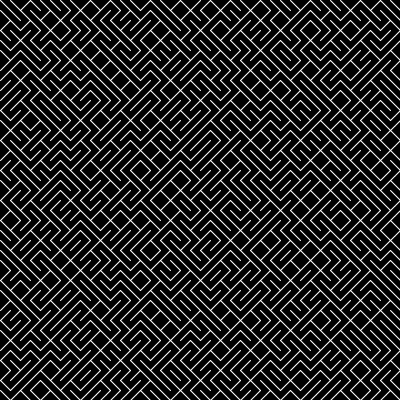# Processing.py: How to implement 10 PRINT

Continuing the Processing.py series, this article demonstrates how to implement ’10 PRINT’ to create interesting line images. 10 PRINT is an old Commodore 64 one-line program written in BASIC that looks like this:

`10 PRINT CHR\$(205.5+RND(1)); : GOTO 10`

Processing.py: How to use shapes, placements, and colors

Here is the Processing.py code to implement 10 PRINT:

```x = 0
y = 0
spacing = 10
rand_point = 0.50

def setup():
size(400, 400)
background(0)
stroke(255)
# stroke('#2C4AF0')  # blue
# stroke('#106A09')  # green
# stroke('#611ED6')  # purple
# strokeWeight(3)

def draw():
global x, y, spacing, rand_point
if (random(1) < rand_point):
line(x, y, x+spacing, y+spacing)
else:
line(x, y+spacing, x+spacing, y)
x += spacing

if x > width:
x = 0
y += spacing

saveFrame("print10_21.gif")```

• The `draw()` function in Processing.py acts like a continuous loop, similar to a Pygame main event loop
• The `spacing` variable controls the length of the diagonal lines
• The `background()` variable controls the color of the background
• The `stroke()` variable controls the color of the lines
• The `strokeWeight()` variable controls the thickness of the lines
• The `rand_point` is the sorting point at which each random number that is generated will be rendered as either a forward slash or a back slash.